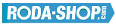# Remote ControlManufacturer Product Image Item Name Price### 606-2RS

Miniature Metric Series Chrome Steel Rubber Seals on Both sides Dimension d=6.00 Dimension D=17.00 Dimension B=6.00 Dinamic Load Rating=2263 Static Load Rating=846 Weight=0.007gr. Speed limit Grease=38.0 Speed limit Oil=45.0
inside diameter = 6.00
outside diameter = 17.00
width = 6.00
€2.24### 606-2Z

Miniature Metric Series Chrome Steel Metallic Shields on both sides Dimension d=6.00 Dimension D=17.00 Dimension B=6.00 Dinamic Load Rating=2263 Static Load Rating=846 Weight=0.007gr. Speed limit Grease=38.0 Speed limit Oil=45.0
inside diameter = 6.00
outside diameter = 17.00
width = 6.00
€2.24### 607

Miniature Metric Series Chrome Steel Open(No Shields) Dimension d=7.00 Dimension D=19.00 Dimension B=6.00 Dinamic Load Rating=2336 Static Load Rating=896 Weight=0.008gr. Speed limit Grease=36.0 Speed limit Oil=43.0
inside diameter = 7.00
outside diameter = 19.00
width = 6.00
€2.24### 607-2RS

Miniature Metric Series Chrome Steel Rubber Seals on Both sides Dimension d=7.00 Dimension D=19.00 Dimension B=6.00 Dinamic Load Rating=2336 Static Load Rating=896 Weight=0.008gr. Speed limit Grease=36.0 Speed limit Oil=43.0
inside diameter = 7.00
outside diameter = 19.00
width = 6.00
€2.24### 607-2Z

Miniature Metric Series Chrome Steel Metallic Shields on both sides Dimension d=7.00 Dimension D=19.00 Dimension B=6.00 Dinamic Load Rating=2336 Static Load Rating=896 Weight=0.008gr. Speed limit Grease=36.0 Speed limit Oil=43.0
inside diameter = 7.00
outside diameter = 19.00
width = 6.00
€2.24### 608

Miniature Metric Series Chrome Steel Open(No Shields) Dimension d=8.00 Dimension D=22.00 Dimension B=7.00 Dinamic Load Rating=3293 Static Load Rating=1379 Weight=0.014gr. Speed limit Grease=34.0 Speed limit Oil=40.0
inside diameter = 8.00
outside diameter = 22.00
width = 7.00
€2.24### 608-2RS

Miniature Metric Series Chrome Steel Rubber Seals on Both sides Dimension d=8.00 Dimension D=22.00 Dimension B=7.00 Dinamic Load Rating=3293 Static Load Rating=1379 Weight=0.014gr. Speed limit Grease=34.0 Speed limit Oil=40.0
inside diameter = 8.00
outside diameter = 22.00
width = 7.00
€2.24### 608-2Z

Miniature Metric Series Chrome Steel Metallic Shields on both sides Dimension d=8.00 Dimension D=22.00 Dimension B=7.00 Dinamic Load Rating=3293 Static Load Rating=1379 Weight=0.014gr. Speed limit Grease=34.0 Speed limit Oil=40.0
inside diameter = 8.00
outside diameter = 22.00
width = 7.00
€2.24### 608-2ZW6

Miniature Metric Series Chrome Steel Metallic Shields on both sides Dimension d=8.00 Dimension D=22.00 Dimension B=6.00 Dinamic Load Rating=3293 Static Load Rating=1379 Weight=0.013gr. Speed limit Grease=34.0 Speed limit Oil=40.0
inside diameter = 8.00
outside diameter = 22.00
width = 6.00
€2.24### 608/24

Miniature Metric Series Chrome Steel Open(No Shields) Dimension d=8.00 Dimension D=24.00 Dimension B=7.00 Dinamic Load Rating=3293 Static Load Rating=1379 Weight=0.025gr. Speed limit Grease=34.0 Speed limit Oil=40.0
inside diameter = 8.00
outside diameter = 24.00
width = 7.00
€2.24